# Modeling Division of a Whole Number by a Fraction Online Quiz

Following quiz provides Multiple Choice Questions (MCQs) related to Modeling Division of a Whole Number by a Fraction. You will have to read all the given answers and click over the correct answer. If you are not sure about the answer then you can check the answer using Show Answer button. You can use Next Quiz button to check new set of questions in the quiz.### Explanation

Step 1: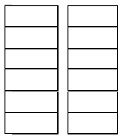2 ÷ $\frac{1}{6}$ = $\frac{2}{1}$ × $\frac{6}{1}$ = 12

Step 2:

2 wholes are being divided into six equal parts each. So two divided by one-sixths = 12

### Explanation

Step 1: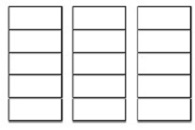3 ÷$\frac{1}{5}$ = $\frac{3}{1}$ × $\frac{5}{1}$ = 15

Step 2:

3 wholes are being divided into five equal parts each. So three divided by one-fifths = 15

### Explanation

Step 1: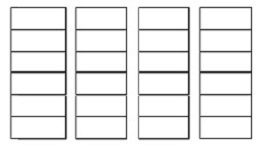4 ÷$\frac{1}{6}$ = $\frac{4}{1}$ × $\frac{6}{1}$ = 24

Step 2:

4 wholes are being divided into six equal parts each. So four divided by one-sixths = 24

### Explanation

Step 1: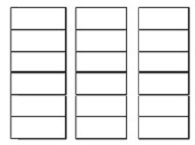3 ÷ $\frac{1}{6}$ = $\frac{3}{1}$ × $\frac{6}{1}$ = 18

Step 2:

3 wholes are being divided into six equal parts each. So three divided by one-sixths = 18

### Explanation

Step 1:4 ÷$\frac{1}{5}$ = $\frac{4}{1}$ × $\frac{5}{1}$ = 20

Step 2:

4 wholes are being divided into five equal parts each. So four divided by one-fifths = 20

### Explanation

Step 1: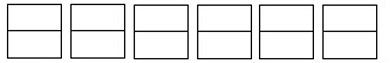6 ÷ $\frac{1}{2}$ = $\frac{6}{1}$ × $\frac{2}{1}$ = 12

Step 2:

6 wholes are being divided into two equal parts each. So six divided by one-halves = 12

### Explanation

Step 1: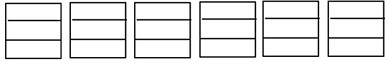6 ÷ $\frac{1}{3}$ = $\frac{6}{1}$ × $\frac{3}{1}$ = 18

Step 1:

6 wholes are being divided into three equal parts each. So six divided by one-thirds = 18

### Explanation

Step 1: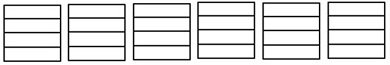6 ÷ $\frac{1}{4}$ = $\frac{6}{1}$ × $\frac{4}{1}$ = 24

Step 2:

6 wholes are being divided into four equal parts each. So six divided by one-fourths = 24

### Explanation

Step 1: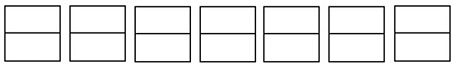7 ÷$\frac{1}{2}$ = $\frac{7}{1}$ × $\frac{2}{1}$ = 14

Step 2:

7 wholes are being divided into two equal parts each. So seven divided by one-halves = 14

### Explanation

Step 1: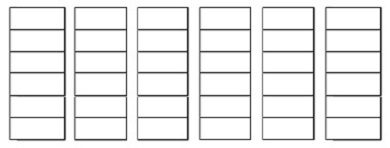6 ÷ $\frac{1}{6}$ = $\frac{6}{1}$ × $\frac{6}{1}$ = 36

Step 2:

6 wholes are being divided into six equal parts each. So six divided by one-sixths = 36

modeling_division_of_whole_number_by_fraction.htm